• 2020-03-19 00:12:59

1.向量点乘公式推导和几何解释

01.向量点乘(dot product)是其各个分量乘积的和，公式：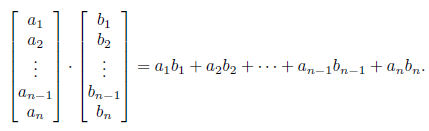用连加号写：

向量a=(a1*e1, a2*e2,a3*e3........an*en)其中e1，e2，e3.......en为正交规范基（俩俩正交，每个向量单位为1）

向量b=(b1*e1, b2*e2,b3*e3........bn*en)其中e1，e2，e3.......en为正交规范基（俩俩正交，每个向量单位为1）

则向量a.b有下面的公式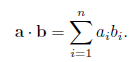02.几何解释：

点乘的结果是一个标量，等于向量大小与夹角的cos值的乘积。

a•b = |a||b|cosθ

如果a和b都是单位向量，那么点乘的结果就是其夹角的cos值。

a•b = cosθ

03.推导过程：

假设a和b都是二维向量，θ1是a与x轴的夹角，θ2是b与x轴的夹角，向量a与b的夹角θ等于θ1 - θ2.

a•b = ax*bx + ay*by

=  (|a|*sinθ1) * (|b| * sinθ2) +   (|a| * cosθ1) * (|b| * cosθ2)

= |a||b|(sinθ1*sinθ2 + cosθ1*cosθ2)

=|a||b|(cos(θ1-θ2))

= |a||b|cosθ

2.点乘交换率和分配率的推导

01.交换率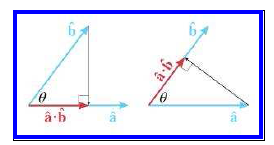02.分配率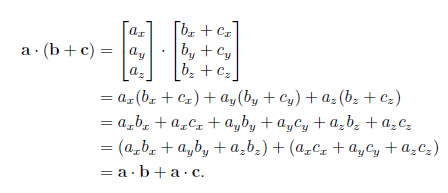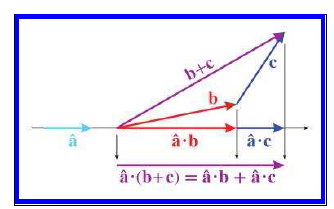注：更多内容参见：<3D math primer for graphics and game development  second edition>点击打开链接

更多相关内容

一般遇到的线性项仅与z轴成线性，因此本文仅讨论线性项与z轴成线性关系的情况，此时，计算式位于(x,y,z)处的项值计算公式变为：

S = S(0) + grad(z)*z                       (2) 以初始化浅埋工况下的自重应力为例，示意见图1，施加的命令流为：
zone initialize stress-zz S(0) grad 0 0 grad(z)zone initialize stress-xx μ*S(0) grad 0 0 μ*grad(z);μ为侧压力系数zone initialize stress-yy μ*S(0) grad 0 0 μ*grad(z)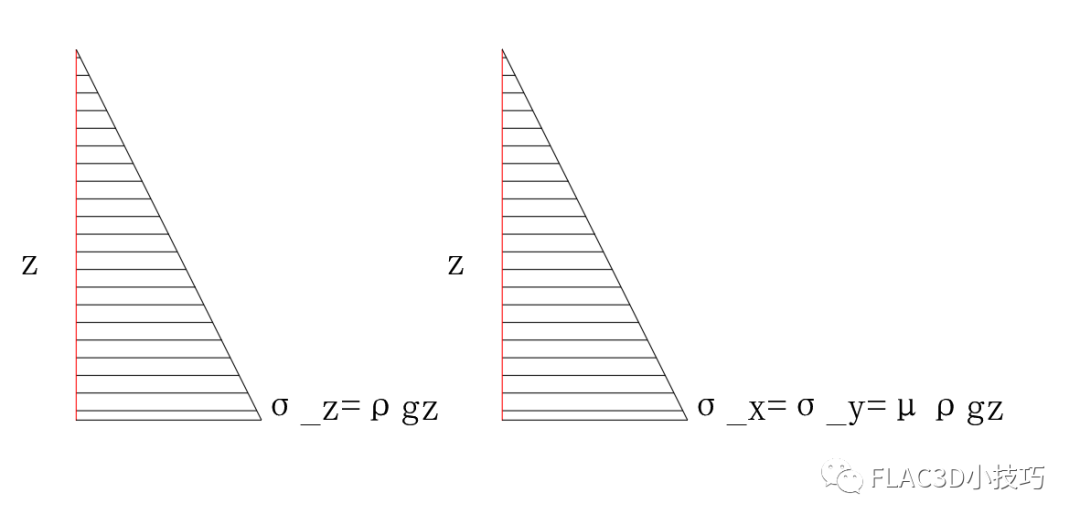图1 浅埋自重应力示意图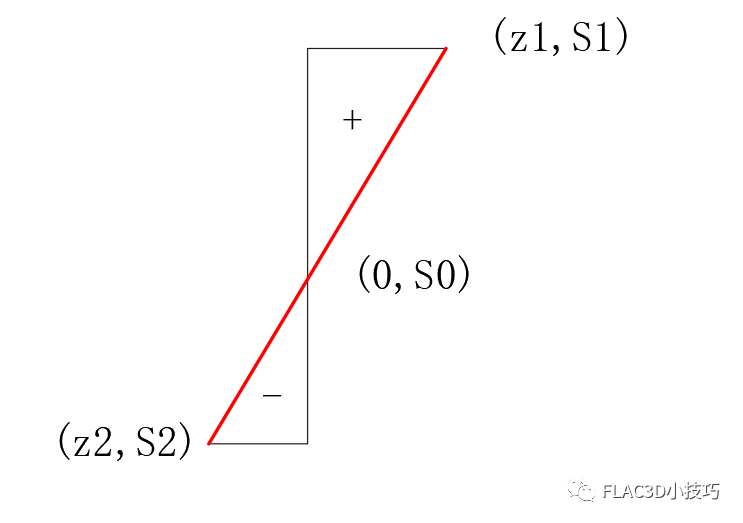图2 梯度计算示意图 由图可知，在计算梯度时，首先需要确定线性荷载两端的两个坐标值；以图1的竖向应力为例，易知位于模型顶端坐标为(0,0)，底端坐标为(z,ρgz)。确定了坐标之后即可求得该线性项关于z的方程：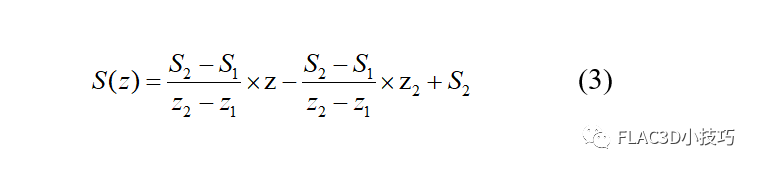由几何关系易知，梯度值grad(z)为方程的斜率，令z = 0 即可求得S(0)。 ======案例演示======

以初始化浅埋工况的自重应力为例(侧压力系数取0.5)，模型尺寸为10m*10m*10m，模型密度取为1000kg/m^3，重力加速度取为10m/s^2。分两个工况模拟，工况1的底面z坐标为0，工况2的底面z坐标为-3。

;模型底面z坐标为0model newzone create brick size 10 10 10zone cmodel assign mohr-coulombzone property bulk 3e8 shear 1e8 cohesion 1e6 friction 15 zone initialize density 1000zone initialize stress-zz -1e5 grad 0 0 1e4zone initialize stress-xx -5e4 grad 0 0 5e3zone initialize stress-yy -5e4 grad 0 0 5e3
运行上述命令流后，模型的三个正应力云图如下：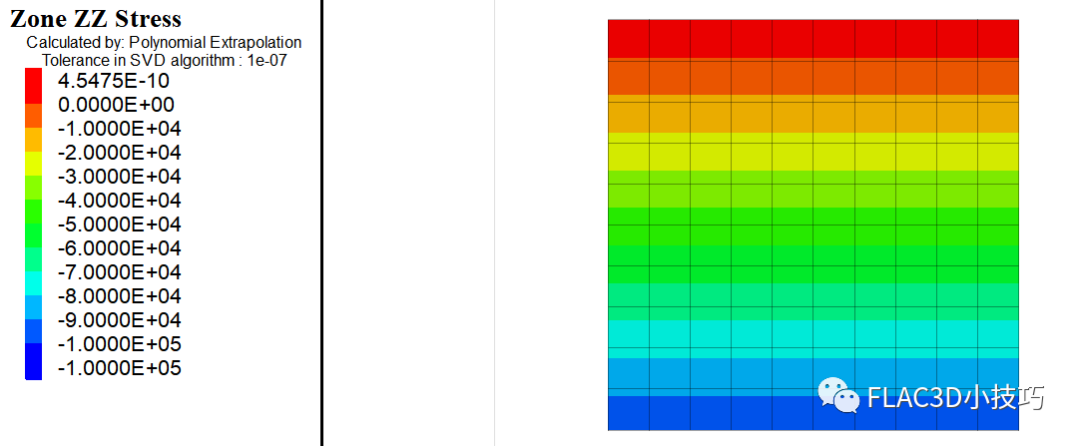图3 stress-zz云图(工况1)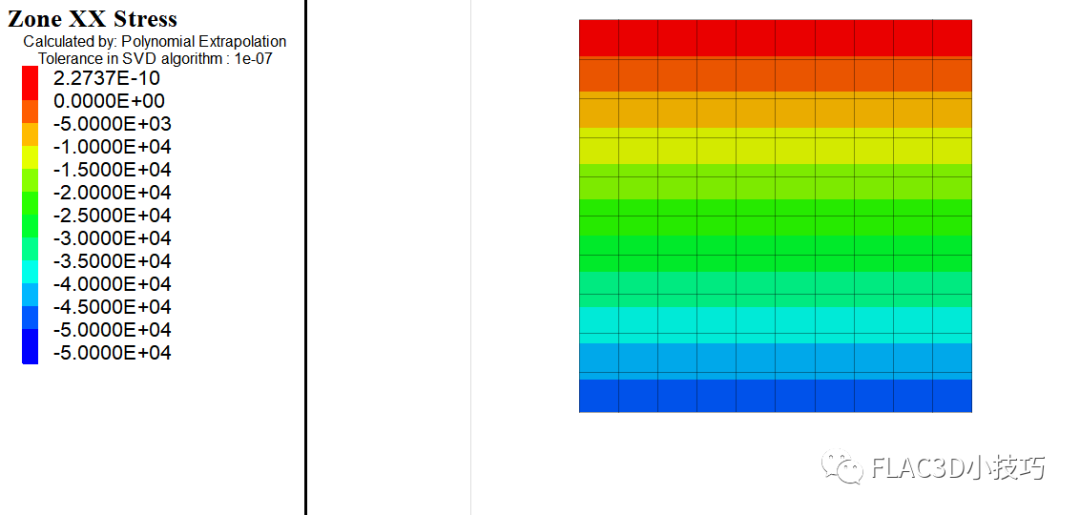图4 stress-xx云图(工况1)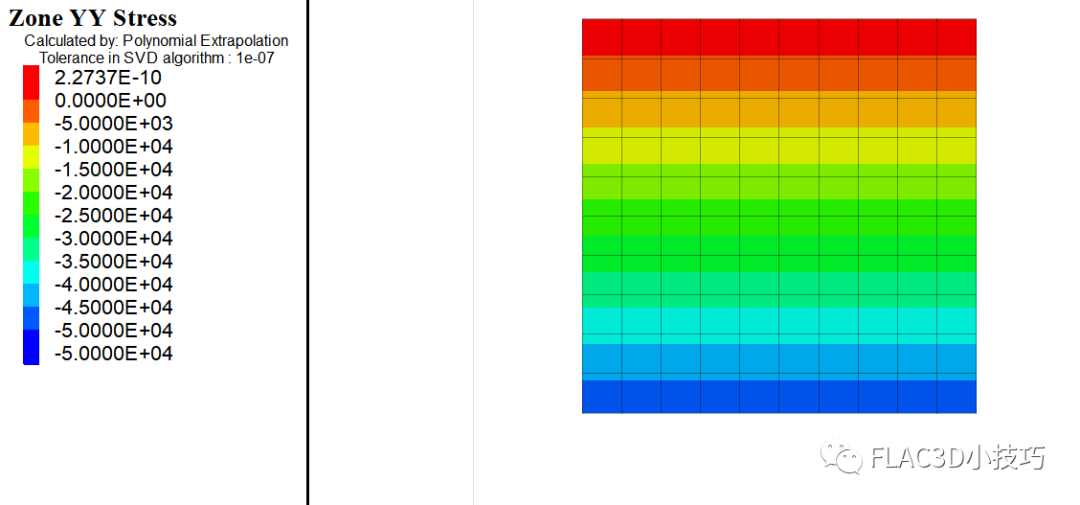图5 stress-yy云图 (工况1 ) 工况2：即z2=-3，z1=7；模型顶部的自重应力为S1=0，底部的自重应力为S2=-1e5Pa。由式(3)计算得到梯度grad = (-1e5-0)/(-3-7)=1e4Pa/m；S(0) = -1e4*(-3)+(-1e5)=-7e4Pa。因此工况2的命令流如下：
;模型底面z坐标为-3model newzone create brick point 0(0,0,-3) point 1(10,0,-3) point 2(0,10,-3) point 3(0,0,7) size 10 10 10zone cmodel assign mohr-coulombzone property bulk 3e8 shear 1e8 cohesion 1e6 friction 15 zone initialize density 1000zone initialize stress-zz -7e4 grad 0 0 1e4zone initialize stress-xx -3.5e4 grad 0 0 5e3zone initialize stress-yy -3.5e4 grad 0 0 5e3

运行上述命令流后，模型的三个正应力云图如下：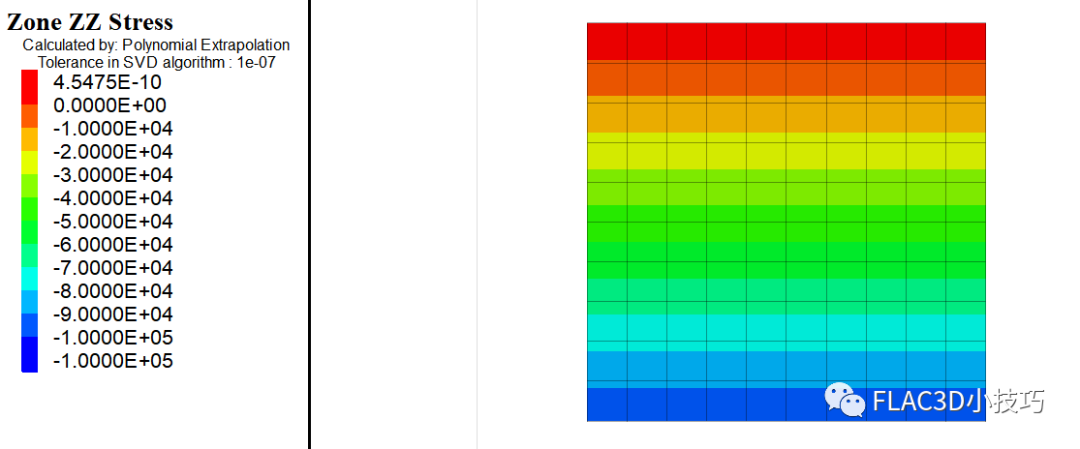图6 stress-zz云图(工况2)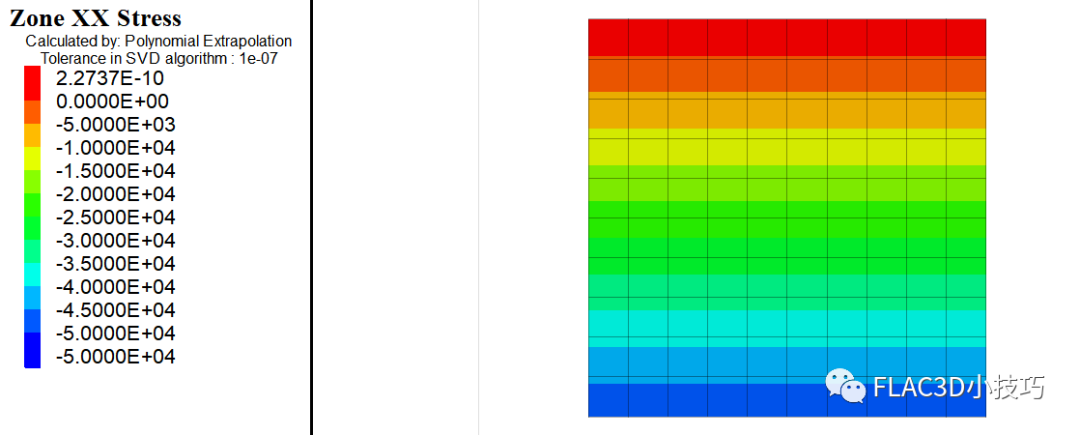图7 stress-xx云图(工况2)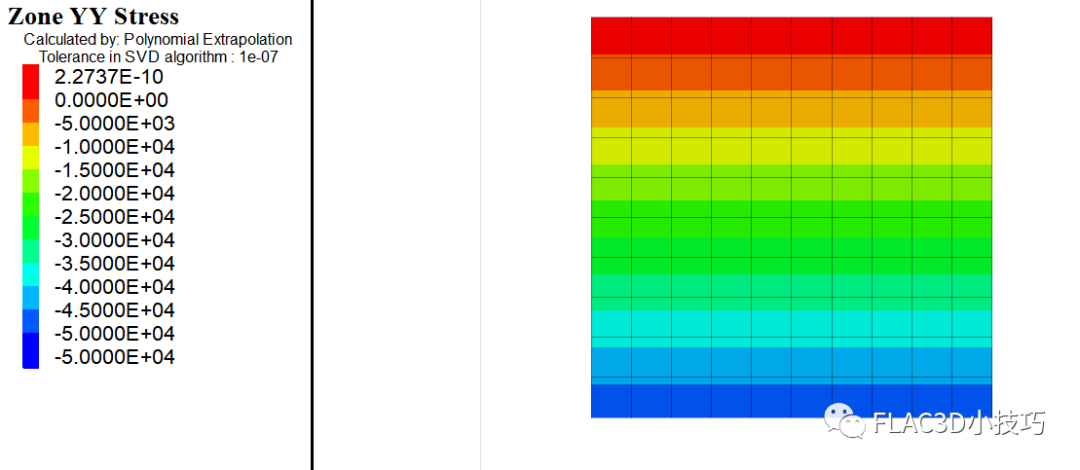图8 stress-yy(工况2)

======总结======

-

展开全文• 模型优化涉及到反向传播过程，反向传播过程需要先推导出梯度计算公式然后利用机器进行代数运算。这篇博文的工作是详细推导了逻辑回归反向传播梯度计算公式(什么是梯度？简单来讲就是成本函数对未知参数向量的导数，...

## 一、简介

在深度学习领域，我们往往采用梯度下降(或上升)法来优化训练函数模型，梯度下降法尤其是在解决凸优化问题上表现极佳。模型优化涉及到反向传播过程，反向传播过程需要先推导出梯度计算公式然后利用机器进行代数运算。这篇博文的工作是详细推导了逻辑回归反向传播梯度计算公式(什么是梯度？简单来讲就是成本函数对所有未知参数所组成向量的导数，梯度是成本函数变化最快的方向)，最后使用标准梯度下降算法来优化逻辑回归并附上逻辑回归优化实现代码。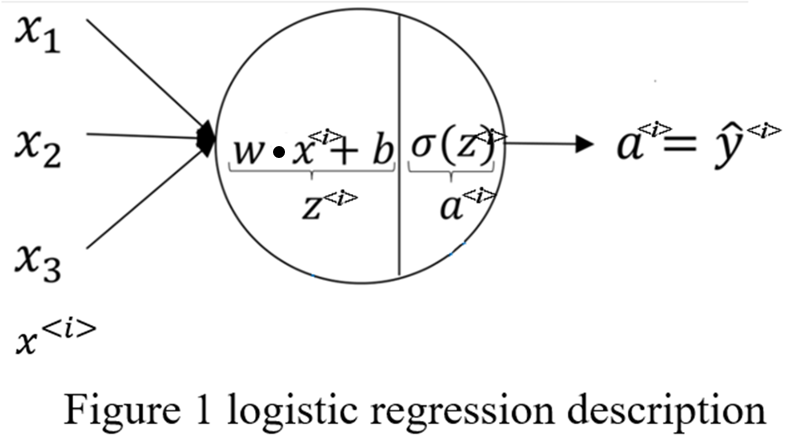逻辑回归问题描述如Figure1所示，它是在多元线性回归的基础上对回归结果使用sigmoid函数进行激活计算得到条件概率 p ( y = 0 ∣ x ) 或 p ( y = 1 ∣ x ) p(y=0|x)或p(y=1|x) ，用来解决二分类问题。这里多说一点如果你想实现多分类可以使用softmax，soft就体现在每一类别都有非零置信度(条件概率)，而不是像hard那样只有一个类别有1置信度其余置信度均为0。以一个样本作为输入的logistic regression forward propagation数学形式化表达为: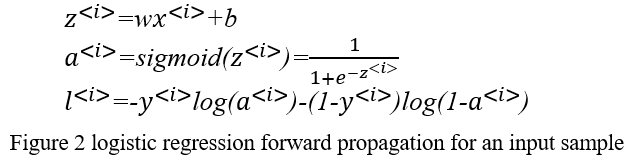## 二、反向传播梯度计算公式推导

接下来是logistic regression back propagation，反向传播梯度计算要求1.熟知前向传播代数计算过程，2.复合函数偏导数的链式法则，3.函数矩阵或函数向量微分理论。在反向传播推导梯度时可以(1)先计算一个输入样本的梯度，然后根据变量与变量的偏导数有相同shape(形式)来推广计算多个输入样本的梯度也就是实现梯度的向量化或叫矩阵化，之所以要将梯度向量化是为了使用相关矩阵理论来简化代数运算。(2)直接对向量化后的多输入样本根据链式法则和向量求导理论推导梯度即可。接下来我将使用这两种方法来详细的推导梯度计算公式。
首先，按方法(1)推导梯度。由于逻辑回归parameters就只有 w w b b ，故而反向传播过程只需计算 ∂ L ∂ w ≡ d w \frac {∂L} {∂w}\equiv dw ∂ L ∂ b ≡ d b \frac {∂L} {∂b}\equiv db ，从而获得梯度 g r a d ( L ) = ( d w , d b ) grad(L)=(dw,db) 。过程如下所示。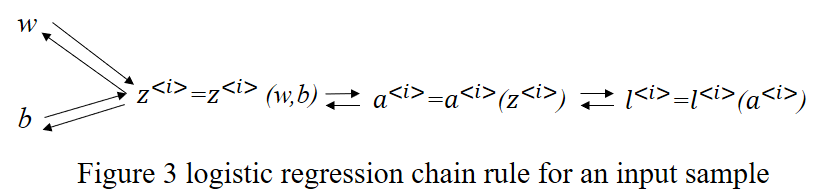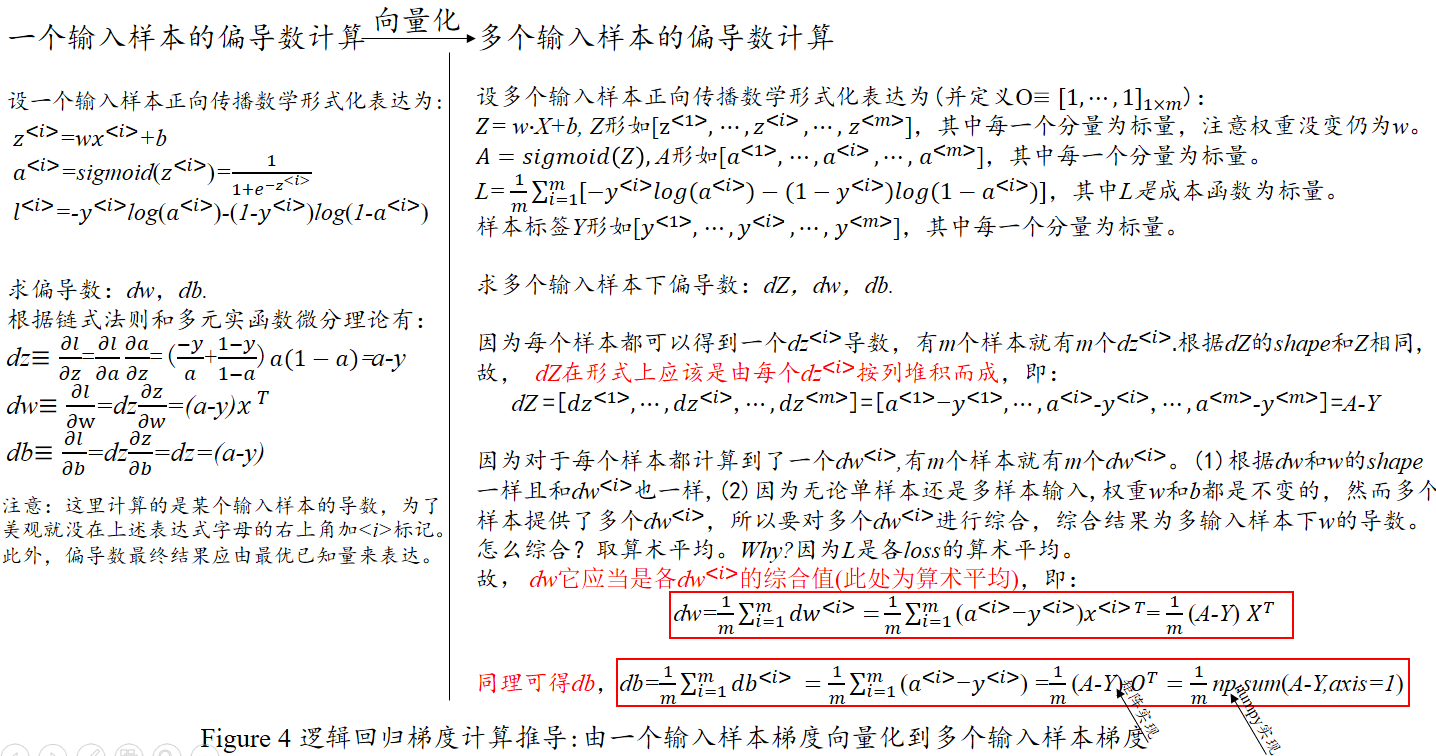其次，按方法(2)计算得到梯度 g r a d ( J ) = ( d w , d b ) grad(J)=(dw,db) ，推导过程如下所示。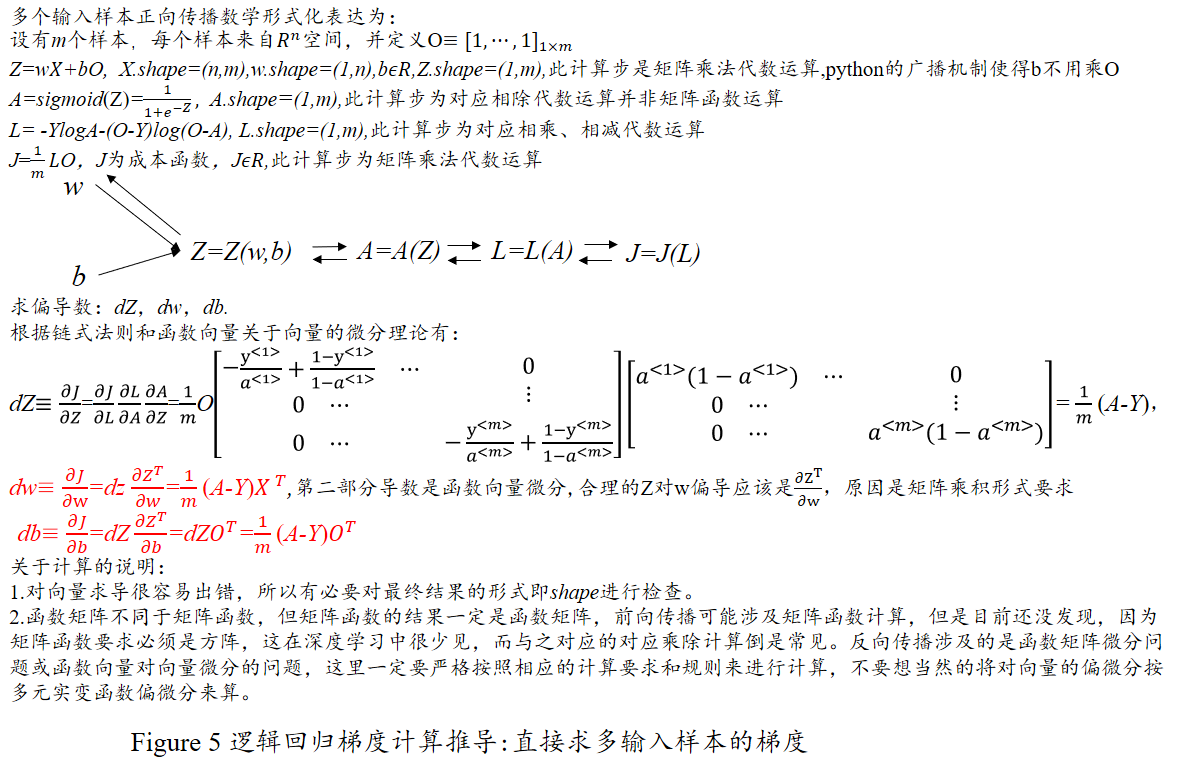## 三、逻辑回归标准梯度下降优化算法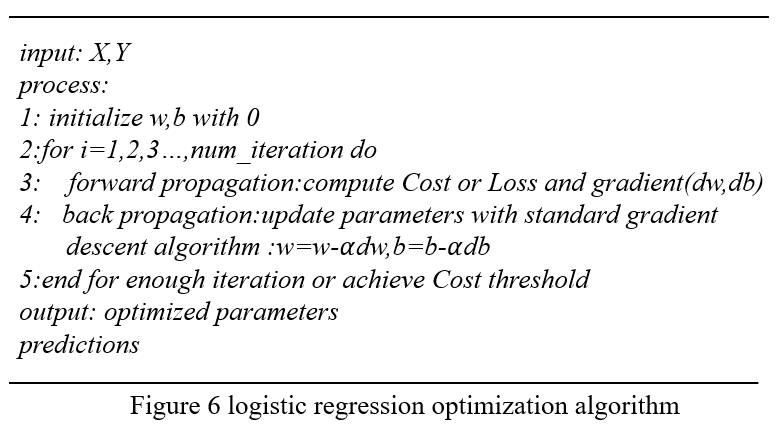逻辑回归优化实现，详见我的github仓库：https://github.com/yiguanxian/implement-logistic-regression 。关于优化算法除了标准梯度下降法还有很多据此法改进的深度学习优化算法，我后面会写一篇关于深度学习中的优化算法的文章，待续…
由于博主水平有限，博文中难免有错误和不当之处，欢迎小伙伴们给与批评和指正，也欢迎小伙伴们补充其他更简单的方法，可以直接留言评论。希望我的这篇博文能对你有所帮助，祝好！

参考：
https://mooc.study.163.com/learn/2001281002?tid=2001392029#/learn/content?type=detail&id=2001702011
https://mooc.study.163.com/learn/2001281002?tid=2001392029#/learn/content?type=detail&id=2001702012
 欧阳光中，朱学炎等.数学分析下册[M].北京：高等教育出版社，2007：168.
吴孟达，李兵等.高等工程数学[M].北京：科学出版社，2004:71-75.

展开全文机器学习 数学
• ## 梯度向量与梯度下降法

万次阅读 多人点赞 2017-10-27 15:37:16

• 偏导数（Partial derivate）
• 方向导数（Directional derivate）
• 线性回归（linear regression）

### 一、方向导数

1，偏导数
先回顾一下一元导数和偏导数，一元导数表征的是：一元函数 f(x) f ( x ) $f(x)$ 与自变量 x x $x$ 在某点附近变化的比率（变化率），如下：

${f}^{\prime }\left({x}_{0}\right)=\frac{\mathrm{d}f\left(x\right)}{\mathrm{d}x}{\mid }_{x={x}_{0}}=\underset{\mathrm{\Delta }x\to 0}{lim}\frac{f\left({x}_{0}+\mathrm{\Delta }x\right)-f\left({x}_{0}\right)}{\mathrm{\Delta }x}$

而二元函数的偏导数表征的是：函数 F(x,y) F ( x , y ) $F(x,y)$ 与自变量 x x $x$ （或 $y$$y$） 在某点附近变化的比率（变化率），如下：

Fx(x0,y0)=Fxx=x0,y=y0=limΔx0F(x0+Δx,y0)F(x0,y0)Δx F x ( x 0 , y 0 ) = ∂ F ∂ x ∣ x = x 0 , y = y 0 = lim Δ x → 0 F ( x 0 + Δ x , y 0 ) − F ( x 0 , y 0 ) Δ x

以长方形的面积 z=F(x,y) z = F ( x , y ) $z = F(x,y)$ 为例，如下图：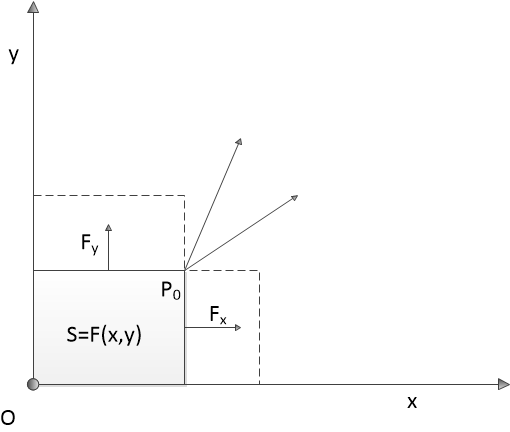如果说 z=F(x,y)=xy z = F ( x , y ) = x ⋅ y $z = F(x,y) = x \cdot y$ 表示以 P(x,y) 点和原点为对角点的矩形的面积，那么 z=F(x,y) z = F ( x , y ) $z = F(x,y)$ P0 P 0 $P_0$ 点对x 的偏导数表示 P0 P 0 $P_0$ 点沿平行于 x 轴正向移动，矩形的面积变化量与 P0 P 0 $P_0$ 点在 x方向的移动距离的比值

Fx(x0,y0)=Fx=limΔx0ΔSΔx F x ( x 0 , y 0 ) = ∂ F ∂ x = lim Δ x → 0 Δ S Δ x

同样地，可得
Fy(x0,y0)=Fy=limΔy0ΔSΔy F y ( x 0 , y 0 ) = ∂ F ∂ y = lim Δ y → 0 Δ S Δ y

需要注意的是：
矩形面积的这个例子有时候也很容易让人混淆，上图中无论 x 还是 y ，都是输入变量，输出变量 S 并没有用坐标轴的形式画出来。也就是说，这个例子实际上是一个三维空间的函数关系，而不是二维平面的函数关系。

向量角度看偏导数：
偏导数向量 [FxFy] [ F x F y ] $\begin{bmatrix}F_x\\ F_y\end{bmatrix}$是坐标向量（原向量） [xy] [ x y ] $\begin{bmatrix}x\\ y\end{bmatrix}$的线性变换，详见《Essence of linear algebra》：求导是一种线性变换。
扩展一下：如果 P0 P 0 $P_0$ 点不是沿平行于 x 轴方向或平行于 y 轴方向，而是 沿与 x 轴成夹角 α α $\alpha$ 的一条直线 l l $l$ 上移动，那么面积的增量与 ${P}_{0}$$P_0$ 点在该直线上移动的距离的比值关系是什么呢？
假设 P0 P 0 $P_0$ 点在这条直线上移动的距离为 Δt Δ t $\Delta t$ ，则有
{Δx=ΔtcosαΔy=Δtsinα { Δ x = Δ t ⋅ c o s α Δ y = Δ t ⋅ s i n α

那么，矩形面积增量与移动距离的增量比值是：
limΔt0ΔSΔt=limΔt0(x0+Δtcosα)(y0+Δtsinα)x0y0Δt=limΔt0y0Δtcosα+x0Δtsinα+(Δt)2cosαsinαΔt=y0cosα+x0sinα lim Δ t → 0 Δ S Δ t = lim Δ t → 0 ( x 0 + Δ t ⋅ c o s α ) ⋅ ( y 0 + Δ t ⋅ s i n α ) − x 0 ⋅ y 0 Δ t = lim Δ t → 0 y 0 Δ t ⋅ c o s α + x 0 Δ t ⋅ s i n α + ( Δ t ) 2 ⋅ c o s α ⋅ s i n α Δ t = y 0 c o s α + x 0 s i n α

与偏导数类似，上式也是一个“一元导数”。事实上，这就是方向导数，可记作
Fl=Fl F l = ∂ F ∂ l

2，矢量描述
从矢量角度来看， P0 P 0 $P_0$ 点沿与 x 轴成夹角 α α $\alpha$ 的一条直线 l l $l$ 移动，这个移动方向可以看作是一个矢量 —— “方向矢量”
$\stackrel{\to }{l}=\left(cos\phantom{\rule{thickmathspace}{0ex}}\alpha ,sin\phantom{\rule{thickmathspace}{0ex}}\alpha \right)$

如果 α=0 α = 0 $\alpha = 0$ ，即沿平行 x 轴方向移动；如果 α=π2 α = π 2 $\alpha = \frac{\pi}{2}$ ，即沿平行 y 轴方向移动。因此，偏导数实际上是方向导数的一种特例。
在回顾一下上一节“二元全微分的几何意义”：用切平面近似空间曲面。这个切平面实际上是由两条相交直线确定的平面，而这两条直线分别是 x 方向的偏导数向量 Px P x → $\vec{P_x}$ 和 y 方向的偏导数向量 Py P y → $\vec{P_y}$ 。很显然，这两个向量不但位于同一个平面，而且相互垂直。那么这两个向量合成的新向量也一定位于这个“切平面”。
注：关于偏导数向量相互垂直，可以很容易从偏导数“切片法”推导出来。
将偏导数合成向量记作 P⃗ =(Fx,Fy)=(Fx,Fy) P → = ( F x , F y ) = ( ∂ F ∂ x , ∂ F ∂ y ) $\vec{P} = (F_x,F_y) = (\frac{\partial F}{\partial x},\frac{\partial F}{\partial y})$ 。如果对偏导数向量和方向向量进行矢量“内积”，则有：
P⃗ l⃗ =Fxcosα+Fysinα=Fl P → ⋅ l → = ∂ F ∂ x c o s α + ∂ F ∂ y s i n α = ∂ F ∂ l

这个内积在数值上刚好等于 l⃗  l → $\vec{l}$ 方向的方向导数。
换句话说：方向导数就是偏导数合成向量与方向向量的内积

从向量角度看：
内积在几何上表示投影，也就是说，方向导数（向量）是原向量在某个方向上的投影的变化率。

3，多元方向导数
从上面的矢量内积出发，将 l⃗  l → $\vec{l}$ 与 y 轴方向的夹角表示为 β β $\beta$ ，则方向导数的公式可以变化为：
Fl=Fxcosα+Fycosβ ∂ F ∂ l = ∂ F ∂ x c o s α + ∂ F ∂ y c o s β

类似地，推广到多维空间
Fl=Fxcosα1+Fycosα2+Fzcosα3+ ∂ F ∂ l = ∂ F ∂ x c o s α 1 + ∂ F ∂ y c o s α 2 + ∂ F ∂ z c o s α 3 + ⋅ ⋅ ⋅

当然，从向量内积的角度更容易得到这个公式
P⃗ l⃗ =(Fx,F,Fz,)(cosα1,cosα2,cosα3,) P → ⋅ l → = ( ∂ F ∂ x , ∂ F ∂ , ∂ F ∂ z , ⋅ ⋅ ⋅ ) ⋅ ( c o s α 1 , c o s α 2 , c o s α 3 , ⋅ ⋅ ⋅ )

### 二、梯度

还是从上面的矩形面积这个例子出发，来探索什么是“梯度”。
假设 P0 P 0 $P_0$ 点的坐标是 (3,1) ( 3 , 1 ) $(\sqrt{3},1)$ ， 则

Fx=1,Fy=3,Fl=cosα+3sinα F x = 1 , F y = 3 , F l = c o s α + 3 s i n α

很明显，方向导数（标量）的大小随 矢量 l⃗  l → $\vec{l}$ 的方向不同而不同。那么，这些方向导数中是否存在一个最大值呢？
答案是肯定的！这就是“梯度”（标量）。
F=gradF=max{Fl} ∇ F = g r a d F = m a x { ∂ F ∂ l }

所以，梯度的第一层含义就是“方向导数的最大值”

2，梯度矢量
梯度的第一层含义是“方向导数的最大值”，那么这个最大值是多少呢？或者说 矢量 l⃗  l → $\vec{l}$ 取什么值时才能找到这个最大值？
还是以矩形面积为例
gradF(3,1)=max{Fl}=max{cosα+3sinα}=max{2sin(π6+α)} g r a d F ( 3 , 1 ) = m a x { ∂ F ∂ l } = m a x { c o s α + 3 s i n α } = m a x { 2 s i n ( π 6 + α ) }

显然， α=π3 α = π 3 $\alpha = \frac{\pi}{3}$ 时，取值最大，此时
l⃗ =(cosα,sinα)=(12,32) l → = ( c o s α , s i n α ) = ( 1 2 , 3 2 )

在比较一下偏导数向量
P⃗ =(Fx,Fy)=(1,3) P → = ( F x , F y ) = ( 1 , 3 )

是不是似曾相识？对的，后者单位化后就变成了前者。
从向量内积的角度来看，更容易理解：
F=gradF=max{Fl}=max{P⃗ l⃗ }=max{P⃗ l⃗ cosθ} ∇ F = g r a d F = m a x { ∂ F ∂ l } = m a x { P → ⋅ l → } = m a x { | P → | ⋅ | l → | ⋅ c o s θ }

很明显， 两个向量的夹角 θ=0 θ = 0 $\theta = 0$ 时，取得最大值。
至此，我们引出了梯度的第二层含义，或者说叫“梯度矢量”
F⃗ =gradF=(Fx,Fy) ∇ F → = g r a d F = ( ∂ F ∂ x , ∂ F ∂ y )

注意：
上面提到的夹角 θ θ $\theta$ 在几何上表示原向量（坐标向量）与导数向量（变化率向量）之间的夹角，所以，梯度的几何含义就是：沿向量所在直线的方向变化率最大。

顺便扩展一下散度（divergence）和旋度（curl）的记号，它们都使用了Nabla算子（微分向量算子），分别如下：
F⃗ ,×F⃗  ∇ ⋅ F → , ∇ × F →

3，举例
总结一下这一节的思路： 偏导数向量合成 $\rightarrow$ 合成向量与方向向量内积 $\rightarrow$ 方向导数 $\rightarrow$ 方向导数的最值 $\rightarrow$ 梯度 $\rightarrow$ 梯度向量与偏导数合成向量相同
转了一圈，又回来了。
从偏导数合成向量到梯度矢量，让我想起了高中物理中的“力的合成与分解”和“沿合力方向做功最有效率”这些物理知识，恰好能与这些数学概念对应上。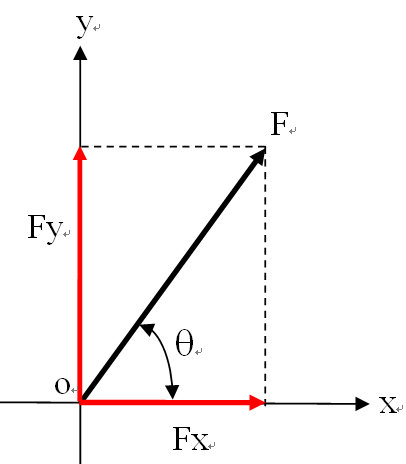如上图，合力表示偏导数合成向量，“垂直分力”表示x方向和y方向的偏导数向量，那么方向向量则对应“做功”路径，而“沿合力方向做功”则表示方向向量与偏导数合成向量重合。Perfect !
注：在重力场或电场中，做功的结果是改变势能，因此，“做功最有效率”又可以表述为“势能变化率最快”。

### 三、梯度下降法

1，梯度下降法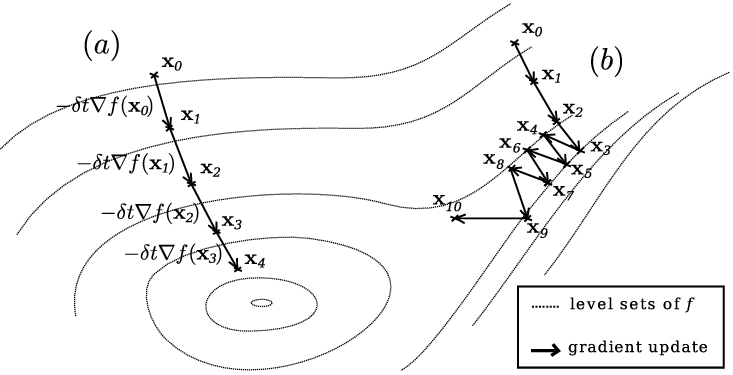看到上面这幅图，你能想到什么？
我想到了两个概念：一是地理学中的“梯田”和“等高线”，下面的链接中，有一篇文章的作者将“梯度下降”形象的比作“从山顶找路下到山谷”，这么看来，等高线肯定与梯度下降有某种关联。
很明显，梯度常常和势能联系在一起，那么势能是什么呢？它就是上图中的弧线圈。这个解释有点虚，给个更贴切的：我们可以把势能看作是 z=f(x,y) z = f ( x , y ) $z = f(x,y)$ 中的这个 z z $z$ ，即函数值。这些弧线圈就表示在它上面的点 $\left(x,y\right)$$(x,y)$ 对应的 z=f(x,y) z = f ( x , y ) $z = f(x,y)$ 的值相等。从空间几何的角度来看这些“弧线圈”，更容易理解： z=f(x,y) z = f ( x , y ) $z = f(x,y)$ 表示空间曲面，用 z=c z = c $z = c$ 这样一个平面去截空间曲面，它们的交线就是“弧线圈”，公式表示为

{z=f(x,y)z=c { z = f ( x , y ) z = c

当然啦，上图是把多个弧线圈画到（投影）了一个平面上。
我们也可以这样来理解“梯度下降法”： 导数表征的是“函数值随自变量的变化率” $\rightarrow$ 梯度是各方向导数中的最大值 $\rightarrow$ 沿 “梯度矢量”移动必定变化最快 $\rightarrow$ 沿“梯度矢量” 反方向下降（减少）最快。

2，梯度下降法求极值
求下列函数的极小值
f(x)=x43x3+2f(x)=4x39x2 f ( x ) = x 4 − 3 ∗ x 3 + 2 ⇒ f ′ ( x ) = 4 x 3 − 9 x 2

# From calculation, it is expected that the local minimum occurs at x=9/4

cur_x = 6 # The algorithm starts at x=6
gamma = 0.01 # step size multiplier
precision = 0.00001
previous_step_size = cur_x

def df(x):
return 4 * x**3 - 9 * x**2

while previous_step_size > precision:
prev_x = cur_x
cur_x += -gamma * df(prev_x)
previous_step_size = abs(cur_x - prev_x)

print("The local minimum occurs at %f" % cur_x)

The local minimum occurs at 2.249965

3，梯度下降法与最小二乘法
“机器学习”中有六个经典算法，其中就包括“最小二乘法”和“梯度下降法”，前者用于“搜索最小误差”，后者用于“用最快的速度搜索”，二者常常配合使用。代码演示如下：

# y = mx + b
# m is slope, b is y-intercept
def compute_error_for_line_given_points(b, m, coordinates):
totalerror = 0
for i in range(0, len(coordinates)):
x = coordinates[i]
y = coordinates[i]
totalerror += (y - (m * x + b)) ** 2

# example
compute_error_for_line_given_points(1, 2, [[3, 6], [6, 9], [12, 18]])

22.0

以上就是用“最小二乘法”来计算误差，当输入为 (1,2) ( 1 , 2 ) $(1,2)$ 时，输出为 22.0 22.0 $22.0$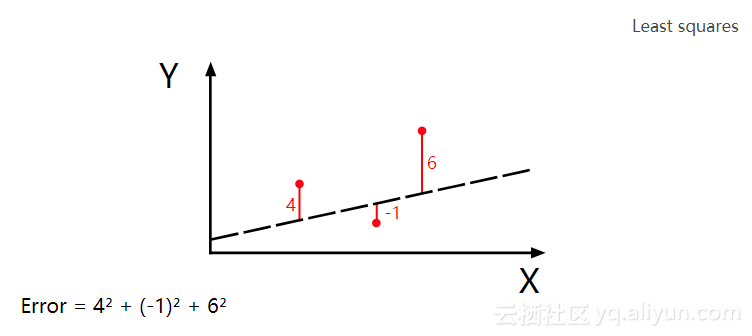很显然，最小二乘法需要不停地调整（试验）输入来找到一个最小误差。而应用“梯度下降法”，可以加快这个“试验”的过程。
以上面这段程序为例，误差是斜率 m 和常数 b 的二元函数，可以表示为

e=g(m,b) e = g ( m , b )

那么，对最小二乘法的参数调优就转变为了求这个二元函数的极值问题，也就是说可以应用“梯度下降法”了。
“梯度下降法”可以用于搜索函数的局部极值，如下，求下列函数的局部极小值
f(x)=x52x32 f ( x ) = x 5 − 2 x 3 − 2

分析：这是一个一元连续函数，且可导，其导函数是：
f(x)=5x46x2 f ′ ( x ) = 5 x 4 − 6 x 2

根据“一阶导数极值判别法”：若函数f(x)可导，且f’(x)在 x0 x 0 $x_0$ 的两侧异号，则 x0 x 0 $x_0$ 是f(x)的极值点。那么，怎么找到这个 x0 x 0 $x_0$ 呢？
很简单，只需要沿斜率（导数值）的反方向逐步移动即可，如下图：导数为负时，沿x轴正向移动；导数为正时，沿x轴负方向移动。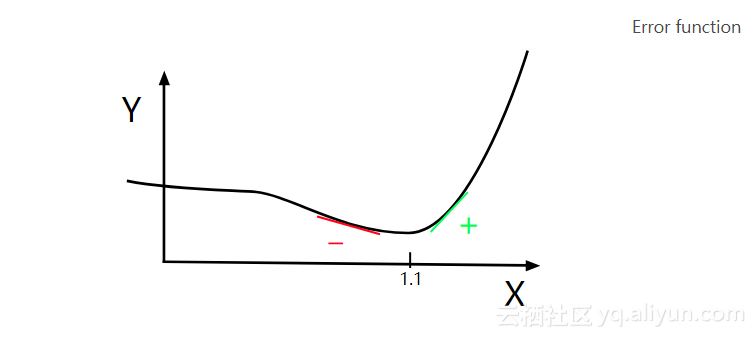current_x = 0.5 # the algorithm starts at x=0.5
learning_rate = 0.01 # step size multiplier
num_iterations = 60 # the number of times to train the function

# the derivative of the error function (x ** 4 = the power of 4 or x^4)
def slope_at_given_x_value(x):
return 5 * x ** 4 - 6 * x ** 2

# Move X to the right or left depending on the slope of the error function
x = [current_x]
for i in range(num_iterations):
previous_x = current_x
current_x += -learning_rate * slope_at_given_x_value(previous_x)
x.append(current_x)   #print(previous_x)

print("The local minimum occurs at %f, it is %f" % (current_x, current_x ** 5 - 2 * current_x ** 3 - 2))

The local minimum occurs at 1.092837, it is -3.051583

import numpy as np
import matplotlib.pyplot as plt
plt.plot(x, marker='*')
plt.show()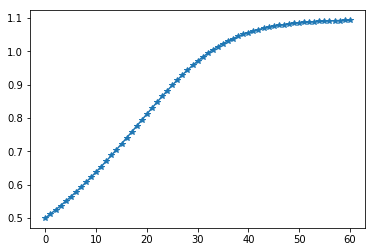沿梯度（斜率）的反方向移动，这就是“梯度下降法”。如上图所示，不管初始化值设为什么，在迭代过程只会越来越接近目标值，而不会偏离目标值，这就是梯度下降法的魅力。
上面这张图是表示的是一个一元函数搜索极值的问题，未必能很好展示梯度下降法的魅力，你再返回去看上面那张“势能梯度图”，那是一个二元函数搜索极值的过程。左边的搜索路径很简洁，而右边的搜索路径，尽管因为初始值的设定，导致它的路径很曲折，但是，你有没有发现，它的每一次迭代事实上离目标都更近一步。我想，这就是梯度下降法的优点吧！
注：这段代码是一元函数求极值，如果是二元函数，则需要同时满足两个分量的偏导数的值为零，下面的线性回归程序算的就是二元偏导数。
通过组合最小二乘法和梯度下降法，你可以得到线性回归，如下：

# Price of wheat/kg and the average price of bread

N = float(len(points))
for i in range(0, len(points)):
x = points[i]
y = points[i]
b_gradient += -(2/N) * (y -((m_current * x) + b_current))
m_gradient += -(2/N) * x * (y -((m_current * x) + b_current))
new_b = b_current -(learningRate * b_gradient)
new_m = m_current -(learningRate * m_gradient)
return [new_b, new_m]

def gradient_descent_runner(points, starting_b, starting_m, learning_rate, num_iterations):
b = starting_b
m = starting_m
for i in range(num_iterations):
b, m = step_gradient(b, m, points, learning_rate)
return [b, m]

gradient_descent_runner(wheat_and_bread, 1, 1, 0.01, 1000)

[3.853945094921183, 2.4895803107016445]

上面这个程序的核心思想就是：在内层迭代的过程中，算出每一步误差函数相当于 m 和 b 的偏导数（梯度），然后沿梯度的反方向调整 m 和 b ；外层迭代执行梯度下降法，逐步逼近偏导数等于0的点。
其中需要注意偏导数的近似计算公式，已知误差函数

E(m,b)=1Ni=0N[yi(mxi+b)]2 E ( m , b ) = 1 N ⋅ ∑ i = 0 N [ y i − ( m ⋅ x i + b ) ] 2

即各点与拟合直线的距离的平方和，再做算术平均。然后可以计算偏导数为
Em=2Nxii=0N[yi(mxi+b)]Eb=2Ni=0N[yi(mxi+b)] ∂ E ∂ m = − 2 N ⋅ x i ⋅ ∑ i = 0 N [ y i − ( m ⋅ x i + b ) ] ∂ E ∂ b = − 2 N ⋅ ∑ i = 0 N [ y i − ( m ⋅ x i + b ) ]

其中的求和公式在程序中表现为内层for循环
下面再给出拟合后的效果图

import numpy as np
import matplotlib.pyplot as plt
plt.plot(a[:,0], a[:,1], 'ro')
x = np.linspace(a[0,0], a[-1,0])
y = m * x + b
plt.plot(x, y)
plt.grid()
plt.show()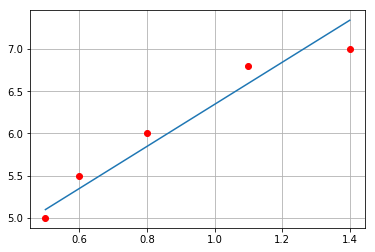对比Numpy

import numpy as np
import matplotlib.pyplot as plt
plt.plot(a[:,0], a[:,1], 'ro')
m, b = np.polyfit(a[:,0], a[:,1], 1)
print([b,m])
x = np.linspace(a[0,0], a[-1,0])
y = m * x + b
plt.plot(x, y)
plt.grid()
plt.show()

[4.1072992700729891, 2.2189781021897814]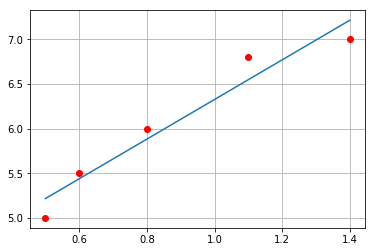展开全文深度学习 机器学习 算法 python
• 神经网络的梯度计算总结 前言 上一篇文章介绍了神经网络需要达到的最终目标，即使所定义的损失函数值达到尽可能的小。那么，是如何达到使得损失函数的值最小的呢？其实，最常使用的核心大招就是“梯度法”进行参数...神经网络 机器学习 python
• ## 图像梯度计算

万次阅读 多人点赞 2018-09-16 11:10:29
连续函数在某一点的导数可以由 得到，图像是离散函数，在某点的梯度可以用向前差商、向后差商或者中心差商获得。这里采用中心差商 可以获取图像某点的导数值。计算过程如下图所示 写成一维卷积的形式等于与如下...机器学习 人工智能 深度学习 算法
• 梯度下降数学解释： 场景假设 梯度下降法的基本思想可以类比为一个下山的过程。 假设这样一个场景：一个人被困在山上，需要从山上下来(找到山的最低点)。但此时山上的浓雾很大，导致可视度很低；因此，下山的路径...
• 算法概述 逻辑回归(Logistic)虽带有回归二字，但它却是一个经典的二分类算法，它适合处理一些二分类任务，例如疾病...利用逻辑回归进行分类的主要思想是：根据现有数据对分类边界线建立回归公式，以此进行分类。 线...python 机器学习 逻辑回归 分类算法
• 1. 批量梯度下降法BGD 2. 随机梯度下降法SGD 3. 小批量梯度下降法MBGD 4. 总结 　在应用机器学习算法时，我们通常采用梯度下降法来对采用的算法进行训练。其实，常用的梯度下降法还具体包含有三种不同的形式，...机器学习 python 算法 深度学习 人工智能
• 向量、矩阵求导，和对标量求导还是有点区别，特别是转置和不转置，在网上参考了其他资料整理一下。 介绍： 在矩阵求导中，分为两种布局：分别是分子布局（Numerator Layout）和分母布局（Denominator Layout）。 ...
• 对神经网络有一定了解的同学知道：一般的神经网络的具体实现都是通过矩阵实现的，包括误差反向传导，梯度计算和更新等等，比如y=w*x+b，这里的所有变量都是矩阵，我们通常会叫w和b为参数矩阵，矩阵相乘首先效率比较...神经网络 batch mini-batch
• ## 方向导数与梯度

千次阅读 2020-12-18 20:53:56
这就需要我们计算温度分布场中各点沿不同方向的温度变化率，从而确定出温度下降的最快方向。沿不同方向的变化率就是方向导数问题，而下降的最快方向就是梯度问题。 顾名思义，方向导数就是某个方向上的导数。 什么是...
• 最近经常会遇到常数和向量向量求导的计算，感觉需要总结点什么了。以后，我还会在这个文档中添加新的公式。 前提和定义 首先做如下定义，已知fff是关于列向量x=[x1x2...xn]T\boldsymbol{x}= [x_{1}\quad x_{2}\...
• ## 梯度下降法和泰勒公式

千次阅读 多人点赞 2019-05-22 19:24:21
第一部分：泰勒公式 在高数中，引出相关需求，其描述如下： 对于一些较复杂的函数，为了便于研究，往往希望用一些简单的函数来近似表达。由于用多项式表示的函数，只要对自变量进行有限次的加，减，乘三种算数运算...数学 机器学习
• RNN梯度详细推导1. RNN的几种常见结构结构一结构二欢迎使用Markdown编辑器新的改变功能快捷键合理的创建标题，有助于目录的生成如何改变文本的样式插入链接与图片如何插入一段漂亮的代码片生成一个适合你的列表创建...机器学习 深度学习 算法
• [转载] ...记得在高中做数学题时，经常要求曲线的切线。...上大学又学习了曲面切线和法向量的求法，求偏导是法向量，然后套公式求出切线。 一个经典例子如下： （来自web上某个《几何应用》ppt...
• ## 梯度下降算法SGD

千次阅读 2018-05-22 14:56:30
因此，梯度是一个向量场。沿着正梯度就可以找到最大值，所谓的梯度上升。沿着负梯度就可以找到最小值，所谓的梯度下降。两者其本质都是一样的。简单公式推导如下：由此可以得到权值更新为（没有引入学习率）：程序...
• 记住公式好办 你先记住哈密顿算子▽ 他表示一个矢量算子（注意）： 　▽≡i*d/dx+j*d/dy+k*d/dz 　运算规则: 　一、▽A=(i*d/dx+j*d/dy+k*d/dz)A=i*dA/dx+j*dA/dy+k*dA/dz 　这样标量场A通过▽的这个运算就...
• 再看了一篇博客后，了解了梯度下降向量化的推导公式，所以便写篇博客记录一下，加深一些记忆。 首先，对于输入矩阵X为n*m的矩阵 所以预测值为y^\widehat{y}y​: MSE=12∗(y^−y)2=12(Xω−y)2\frac{1}{2} *(\...
• ## 密度梯度理论

千次阅读 2020-07-10 15:42:33
密度梯度限制理论–半导体器件仿真 一、静电和电荷载流子守恒 半导体器件的物理场仿真包括由电场（漂移）和载流子浓度梯度（扩散）驱动的载流子迁移。电场（V/m）由准静态假设下的静电方程式给出： 式中， 是介电...
• 在2.1中提到的梯度下降，为了计算出参数的梯度，需要代入整个数据集，这样一次更新计算量非常大，因此提出随机梯度下降方法，即每一个更新都是从数据及中随机抽样部分数据（batch), 在词向量计算中对每一个window...
• 对于很多数学和工程问题，我们常常需要使用到梯度、散度和旋度方程，而有的时候，虽然在使用这些方程，却对他们其中的物理意义不甚清楚，这样的后果是只能对公式死记硬背，但结果还是常常忘记。这篇文章便从这三大...math coordinate gradient
• 很久以前看过循环神经网络的相关知识，但一直没有推梯度。这次仔细的看了一遍梯度推导。关于循环神经网络的前向理论，http://blog.csdn.net/juanjuan1314/article/details/52020607 这一篇译文已经有详细的写过了。...循环神经网络
• NN这块的公式，前馈网络是矩阵乘法。损失函数的定义也是一定的。 但是如何更新参数看了不少描述，下面的叙述比较易懂的：   1、在吴恩达的CS229的...第二步：通过对误差比重（a,b）求导计算出误差梯度（注：Y...
• 计算x,y梯度 梯度方向操作 计算x,y梯度 让我们仔细看看梯度方向，特别是它们如何计算和表示。 % Gradient Direction &gt;&gt; pkg load image; &gt;&gt; % Load and convert image to double ...
• 利用微分求梯度的方法计算量太大，而误差反向传播算法的出现提高了计算效率，误差反向传播算法主要基于链式法则,主要有以下几条规则。 1.如果是由a + b = y，则反向传播时a b 的梯度相等，且等于y的梯度 2.如果是a ...
• 黄海广博士在（吴恩达）机器学习课程练习3（ML-Exercise3）中，重写逻辑回归中梯度函数的实现，改为完全向量化（即没有“for”循环） 向量化前的梯度函数（“for”循环模式）： def gradient_with_loop(theta, X, y,...
• 注意(下划线(_)后面的字符是下标) 前向传播推导 门的计算公式 状态的计算公式 以遗忘门为例说明导数的计算。我们需要遵循下图中红色箭头的路径。 我们画出一条从f_t到代价函数J的路径，也就是 f_t→C_t→h_t→J。 ...深度学习 神经网络 LSTM...